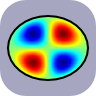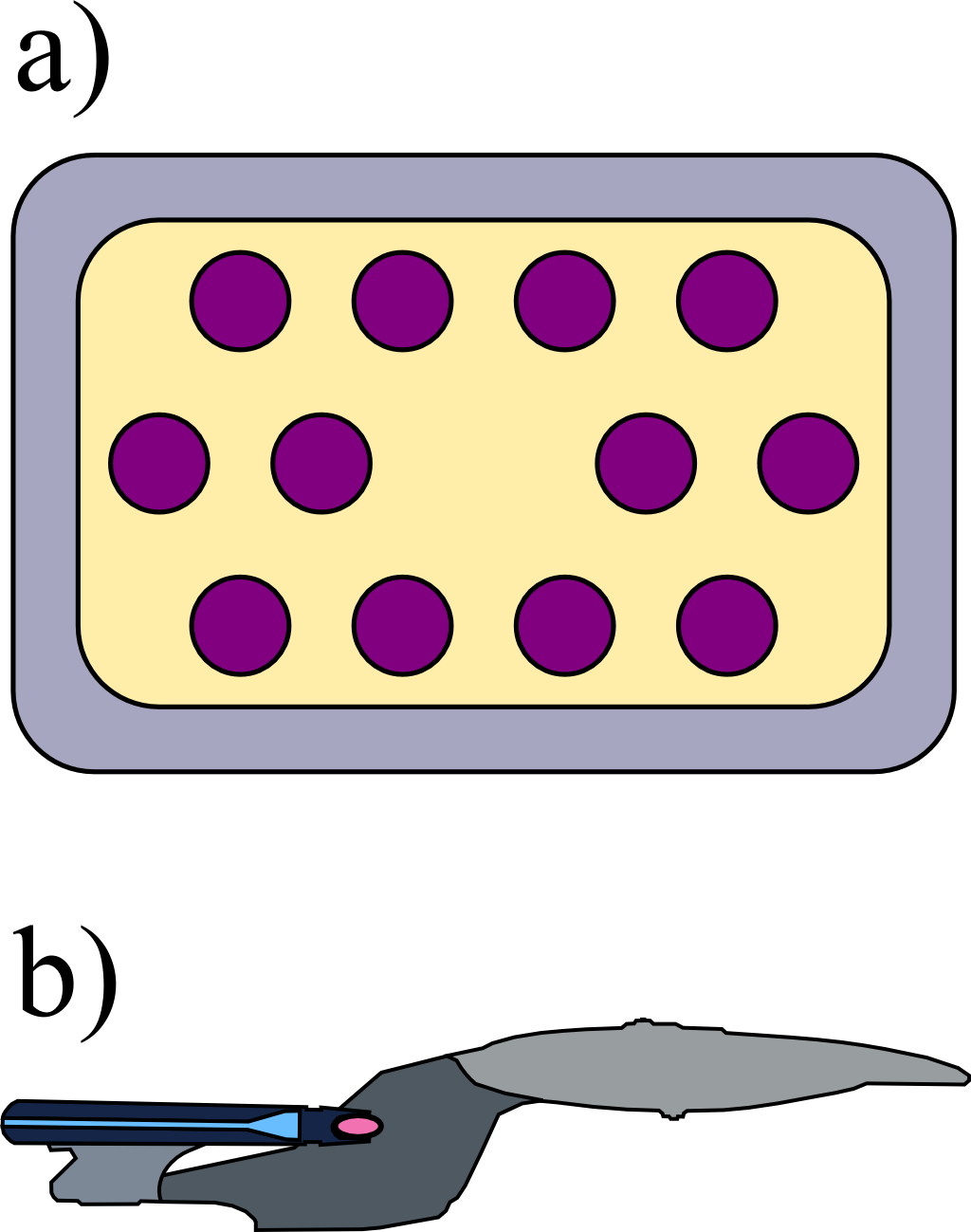The Boundary Integral EquationThe so-called boundary integral equation relates the values of the electrostatic potential in some domain to its values at that domain's boundary. In this problem we will derive this important statement which leads to the "Boundary Element Method", a discretized version with numerical applications throughout science and engineering.

Problem Statement

Derive the boundary integral equation for a region $$\Omega$$ containing no charges:

1. Given the Poisson equation for a function $$u\left(\mathbf{r}\right)$$, $$\Delta u\left(\mathbf{r}\right)=0$$ in some domain $$\Omega$$ and likewise for $$w\left(\mathbf{r}\right)$$, derive Green's second identity $\int_{\Omega}u\left(\mathbf{r}\right)\Delta w\left(\mathbf{r}\right)dV-\int_{\Omega}w\left(\mathbf{r}\right)\Delta v\left(\mathbf{r}\right)dV = \int_{\partial\Omega}\left[u\left(\mathbf{r}\right)\partial_{\boldsymbol{\mathbf{\nu}}}w\left(\mathbf{r}\right)-w\partial_{\boldsymbol{\mathbf{\nu}}}u\left(\mathbf{r}\right)\right]d\mathbf{A}\ .$
2. Now derive the actual boundary integral equation: Green's function for the Laplace operator is defined by $$\Delta G\left(\mathbf{r},\mathbf{r}^{\prime}\right)=\delta\left(\mathbf{r}-\mathbf{r}^{\prime}\right)$$. Apply Green's second identity now for the electrostatic potential $$\phi\left(\mathbf{r}\right)$$ and $$G\left(\mathbf{r},\mathbf{r}^{\prime}\right)$$.
3. Regarding the boundary integral equation, can you imagine what kind of problem may arise if one uses it in a numerical implementation?

Background: The Boundary Element MethodUsing Green's second identity, the boundary integral equation relates the values of the electrostatic potential inside some domain $$\Omega$$ to its values at the boundary of $$\Omega$$. This is per se not really useful  because we might be able to study just a single dielectric body in some embedding medium. However, the approach can be generalized to several domains, i.e. more complex bodies - examples for such bodies can be seen on the left: a) a photonic crystal embedded in some metal, b) a (much) more sophisticated structure.

The generalization is done using the appropriate boundary conditions between adjacent domains. This leads to a system of coupled boundary integral equations which can be discretized replacing the integrals by sums. In turn, the system of boundary integral equations can be evaluated on a computer - the numerical approach is called “Boundary Element Method”. It has a lot of applications in optics, acoustics, aerodynamics since one can use this this method also for other kinds of wave equations. Within the Boundary Element Method, several scientific and engineering questions can be cast into questions on the arising system of equations, hence properties of some matrix. For instance, one can calculate the response of a dielectric body to a giving excitation or a direct computation of the resonances. A lot of books and papers exist. For a realization with an optics/nanophotonics background, see J. Wierig's excellent “Boundary element method for resonances in dielectric microcavities”, Journal of Optics A: Pure and Applied Optics 5, 2003.